# Normal Distribution

Published:

This post covers Normal Distribution from https://www.mathsisfun.com/data/standard-normal-distribution.html and https://www.mathsisfun.com/data/standard-deviation.html

Ztable: Z

# Data Distribution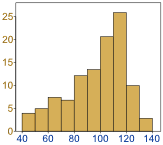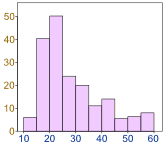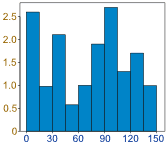• Examples of Normal Distribution

• Heights of people
• size of things produced by machines
• errors in measurements
• blood pressure
• marks on a test

## Normal Distribution

• mean = median = mode
• 50% of values less than the mean and 50% greater than the mean## Standard Deviation

• measure of how spread out numbers are
• square root of the Variance
• Variance is average of the squared differences from the Mean

• Example
•• Heights: 600mm, 470mm, 170mm, 430mm and 300mm
• Compute Mean, the Variance, and the Standard Deviation
• Mean
• 394
•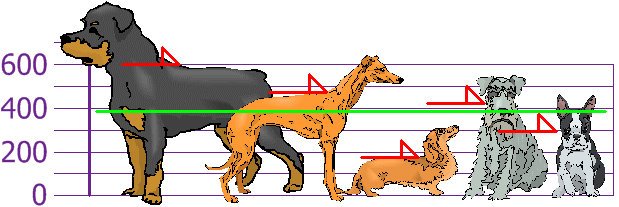• Variance
• Each Dog’s Difference from the mean
•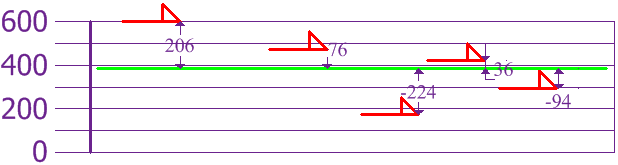• 21704
• Standard Deviation
• 147.32
•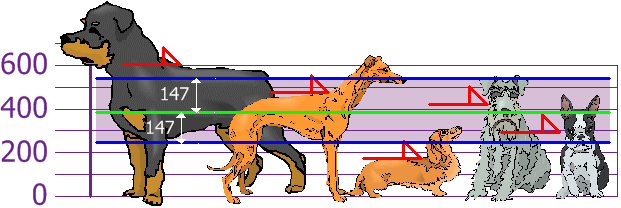• SD is useful since we can show which heights are within one Standard Deviation (147) of the mean (394 mm)
• Using Standard Deviation, we have a standard way of knowing what is normal and what is extra large, or extra small
• Correction for Sample Data
• If the data is population, then variance is average of squared differences
• If the data is sample from a bigger population, we divide by N-1 for calculating variance
• Sample Variance: 27130
• Sample Standard Deviation: 165

# Standard Deviations68% of values are within 1 standard deviation of the mean / 95% of values are within 2 standard deviation of the mean / 99.7% of values are within 3 standard deviation of the mean
• Example
• 95% of students are between 1.1m and 1.7m tall. Assume data is normally distributed, compute mean and standard deviation

• Mean is halfway between 1.1m and 1.7m
• Mean = (1.1m + 1.7m) / 2 = 1.4m
• 95% is 2 standard deviations either side of the mean (a total of 4 standard deviations) so:
• $1~SD = \frac{1.7m-1.1m}{4}=0.15$
• ResultIt is good to know the standard deviation, because we can say that any value is:

• likely to be within 1 standard deviation (68 out of 100 should be)
• very likely to be within 2 standard deviations (95 out of 100 should be)
• almost certainly within 3 standard deviations (997 out of 1000 should be)

## Standard Scores

• The number of standard deviations from the mean is also called

• Standard Score
• sigma
• z-score
• Example

• One student is 1.85m tall

• Is there a standard way of telling information about height• 1.85m is 3 standard deviations from the mean of 1.4
• $\frac{1.85 - 1.4}{.15} = \frac{.45}{.15}=3$
• Thus, z-score is 3.0
•• Example: Travel Time

• 26, 33, 65, 28, 34, 55, 25, 44, 50, 36, 26, 37, 43, 62, 35, 38, 45, 32, 28, 34

• Mean is 38.8 minutes, and the Standard Deviation is 11.4 minutes

• Compute z-scores

• $z = \frac{x - \mu}{\sigma}$
• $z = \frac{x - mean}{std}$
  data = np.array([26, 33, 65, 28, 34, 55, 25, 44, 50, 36, 26, 37, 43, 62, 35, 38, 45, 32, 28, 34])

mean = np.mean(data)
std = np.std(data)

z = (data - mean)/std # stats.zscore(data)
print(z)

z1 = data[np.where(np.abs(z)>1)]
z2 = data[np.where(np.abs(z)>2)]
z3 = data[np.where(np.abs(z)>3)]

print(z1)
print(z2)
print(z3)

• Why Standardize?

• Marks out of 60
• 20, 15, 26, 32, 18, 28, 35, 14, 26, 22, 17
• Most student have less than 30 marks
• Mean = 23 and Standard Deviation = 6.6
• -0.45, -1.21, 0.45, 1.36, -0.76, 0.76, 1.82, -1.36, 0.45, -0.15, -0.91
• Only two students have lower marks than one SD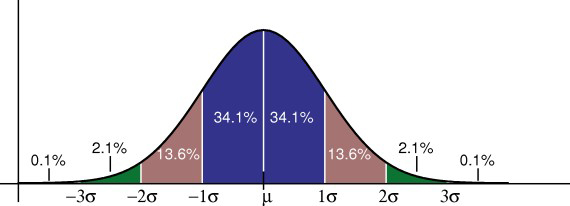• Your score in a recent test was 0.5 standard deviations above the average, how many people scored lower than you did?
• Between 0 and 0.5 is 19.1%
• Less than 0 is 50% (left half of the curve)
• So the total less than you is:
• 50% + 19.1% = 69.1%
• https://www.mathsisfun.com/data/standard-normal-distribution.html
• https://www.statisticshowto.com/probability-and-statistics/normal-distributions/